﻿ 6.8 Application to a Futures Exampleoffice (412) 9679367
fax (412) 967-5958
toll-free 1 (800) 214-3480

D.3  Ito's Lemma

 H

n this topic, we apply the theory developed in Topic 6.7 to an actual hedging example. Recall that initially, the arbitrage-free present value of the futures price is zero, but as subsequent shifts occur in interest rates, this is no longer the case. Here we consider the case of shifts in interest rates that occur subsequently to the time of settlement of the futures contract.

Here we assume that X is the price of some asset, and that price changes are described by the following general diffusion process: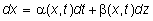Let f(x,t) be the value at time t of any derivative security defined on x (such as a call option).  Here we show that the derivative price, f,  also follows a diffusion process: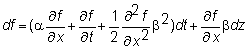This is an important characterization because it describes precisely how the common underlying source of uncertainty, dz, affects both asset and derivative prices.  Looking ahead, this enables a riskless hedge to be designed between the asset and its derivative that eliminates dz, and the value of the derivative to be derived from this hedge.

An intuitive proof of the derivative price dynamics can be obtained by taking a second-order Taylor's series expansion of f(x,t) around a point (x0, y0).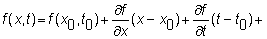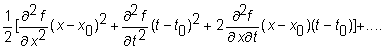Now, substitute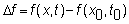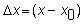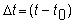and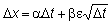to get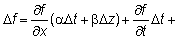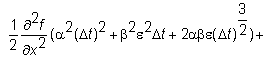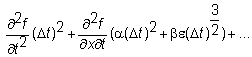As  Dt approaches dt, all terms involving (Dt)2  and (Dt)3/2 go to zero, as do any higher-order terms.  The critical part of the proof then shows that as Dt goes to zero, the term  b2e2Dt becomes non-stochastic and converges to b2dt.  To see that the Dt term does not go to zero, we can write the discrete time analogue of Dx as

.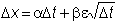Therefore,  (Dx)2 retains Dt, which approaches dt as  Dt goes to zero.

With this, the formula simplifies to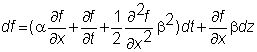which is known as Ito's Lemma.  It says that if f is a function of x and t, then f inherits the stochastic properties of x, and the drift rate and volatility have to be adjusted as given by the lemma.  In particular, df is also normally distributed.

For the stock price, we have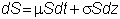Substituting for x, a and  b in the df equation yields: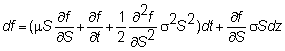This is the general expression for the change in a derivative's price given the stock price process.

In the next topic, we apply Ito's Lemma to derive the Black-Scholes Partial Differential Equation.  This equation expresses the relationship between the “unknown” option valuation function and some of its partial derivatives.